## Motivation

There is an interesting publicly available dataset that has become a bit of a hobby project for me. The data takes some cleaning and restructuring which we may describe in the future. The interesting thing is that a largish set of math and reading items has been administered to a very diverse sample of students, ranging from the final grade of primary education (11-12 year olds), secondary (15-18 yo) and tertiary education (18-19 yo), 14369 persons and 280 items in total. There was some differentiation in that the easiest items were not administered in the highest grades and vice versa. But, as you can imagine, rather than showing a univariate smooth and steady increase of ability through the educational system, this resulted in the motherload of all DIF, not unlike PISA. We will use the mathematics part of this dataset.

I wanted to try a factor analysis to see if there were any interesting differences in the underlying make-up of ability between the sectors. Unfortunately, there are no item properties available so we don’t know which items are about geometry or algebra, if there was or was not a context, a graph, etcetera. Therefore we are left with only the possibility for an exploratory factor analysis. Because the data is very incomplete, we need to use an IRT model. The best known option is mirt (Chalmers 2012). Before trying a multi factor model I decided to try a one factor solution to see how well that went. Factor analysis with just one factor is equivalent to the standard 2PL model, which I could compare against our own package dexterMML. I noted some differences in the estimates. These point to one of the difficulties one can encounter when calibrating a 2PL that merit a further look, which is what this story will be about.

## Minorization and the 2PL

Fitting a 2PL on this dataset takes 2.2 seconds in dexterMML and 22:17 minutes in mirt, but we know dexterMML is relatively fast for incomplete data and in this case every student only did about 10% of the items. Still, the difference is several orders of magnitude and a little concerning since the main cause seems to be that mirt failed to converge.

If we look at the resulting item parameters, we also see a difference: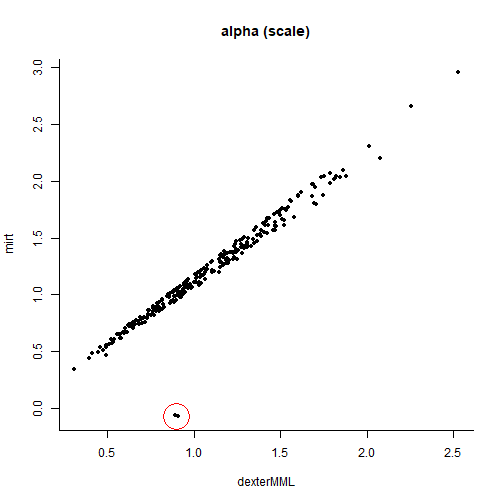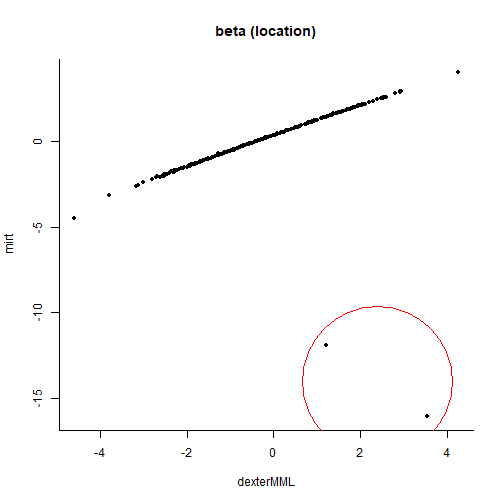For all items but two, mirt and dexterMML seem to agree quite well. But what’s going on with those two items? First let’s look at the fit plots of the offending items.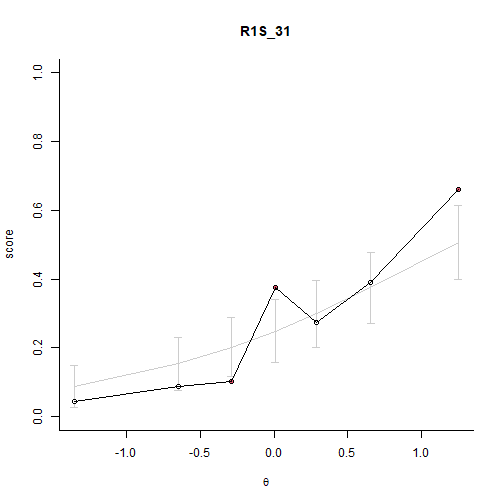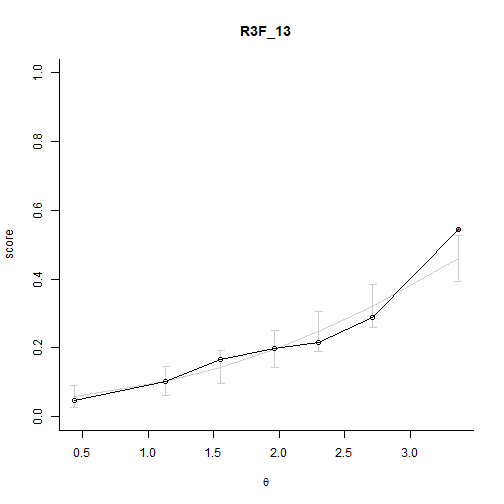That seems OK. How about if we compare the classical Test and item analysis then:

Classical statistics, averaged over booklets, and IRT estimates
TIA
dexterMML
mirt
item_id pvalue rit rir n_persons alpha beta alpha beta
R1S_31 0.28 0.44 0.28 482 0.91 1.2 -0.08 -12
R3F_13 0.22 0.33 0.26 1171 0.89 3.5 -0.07 -16

The two items seem a tad difficult, but a negative discrimination seems clearly wrong. We are inclined to believe the about average discrimination indicated for these two items by dexterMML.

So, what’s going on? The short answer is that this is a general problem that may occur in 2PL estimation, namely getting stuck at a local maximum of the likelihood rather than a global maximum.

The long answer demands slightly more explanation and introduction. In a 2PL marginal model the usual method of estimation is the Expectation Maximization (EM; Dempster, Laird, and Rubin 1977) algorithm. This is a general recipe for maximizing an intractable likelihood function. It consists of two steps:

1. Expectation step. We define a function Q as the expected value of the log likelihood as a function of new estimates of the item and population parameters, based on summary statistics which we compute using the previous parameter estimates and the response data.

2. Maximization step. We find the parameters for the items and population(s) that maximize Q.

And repeat until some stopping criterium is reached. Dempster, Laird, and Rubin (1977) proved that this algorithm is guaranteed to improve the likelihood in each step and so will eventually converge to the optimal solution, although at an increasingly slower pace in each iteration.

Unless, of course, something goes wrong.

The issue we’re currently dealing with is probably related to the second step, the maximization. The Q function has many parameters, but usually in IRT software it can be updated one item at a time, independent of the other items1.

For a single dichotomous 2PL item this is a function with two inputs and one output and the task is to find the two input values for which the output is maximized. Let’s plot this likelihood-related function for our problematic items. We of course use the Q function in dexterMML so the Q function in mirt might be different.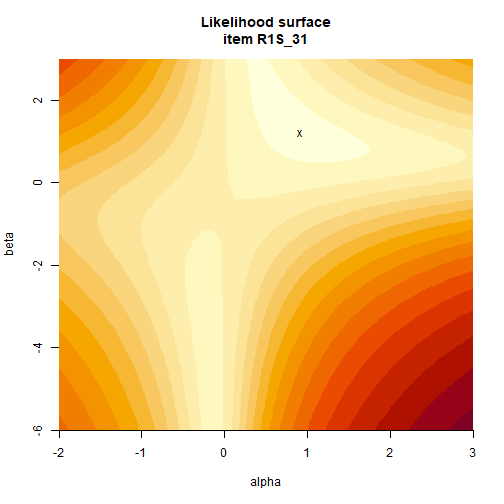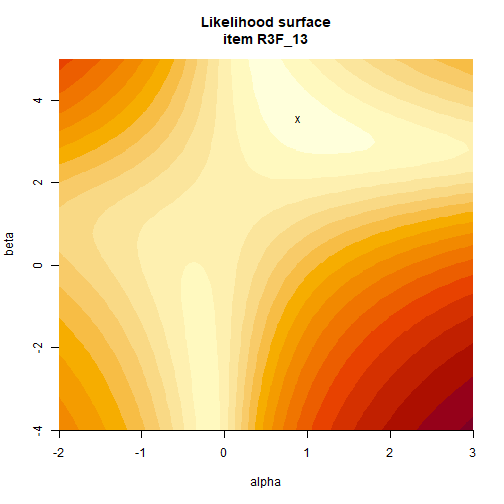These are the functions we need to maximize (in the plot, find the lightest region) for each item. A dichotomous 2PL item always has two local maxima, one with a negative discrimination and one with a positive discrimination. The two plots are rather normal for dichotomous 2PL items. For polytomous items, there is no adequate graphical representation, but they might have more than 2 local maxima.

So, the problem is, a minorization2 algorithm can get stuck at a local optimum. To see how this works, we have to understand what a minorization algorithm is. Informally, a minorization algorithm finds a local minimum by evaluating a function a finite number of times. There are many optimization algorithms, several of which are included with R. A ‘better’ algorithm needs fewer function evaluations, since evaluations of functions are potentially time-consuming. Some algorithms just evaluate the function to be minimized, others need its first or higher order derivatives as well.

But the operative word here is local minimum. There are no algorithms that can determine a global minimum for any unknown function3. This means that whether the algorithm arrives at the global minimum or a local minimum depends on the starting point (and the algorithm).

We can easily see the effect of the starting values by running a Minorization function on our likelihood. DexterMML uses the Dennis and Schnabel algorithm (Dennis and Schnabel 1983), used in R’s nlm() function. We will try that one first. We take only one of the aforementioned items since the two are very similar. We use alpha=1.5, beta=2.5 and alpha=-1, beta=-1 as starting values and we see that we arrive at two minima which look like the resulting parameters from dexterMML and mirt respectively. So we now have a decent idea what’s going on.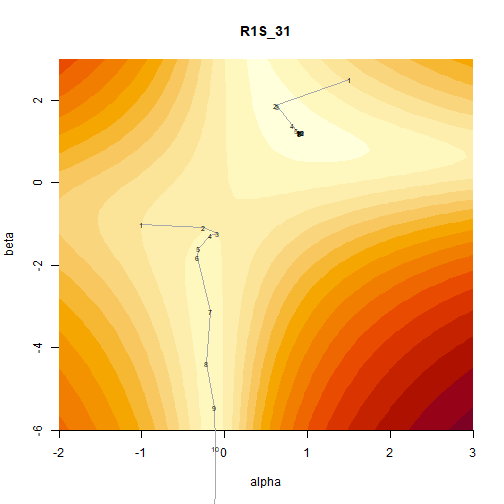We can think of a number of possible solutions and the plot above immediately points to one. What if, for example, we always run the algorithm twice, starting once in the upper-right quadrant and once in the lower left quadrant and take the best result? Wouldn’t we always get the optimal result? It’s not a bad idea, let’s try: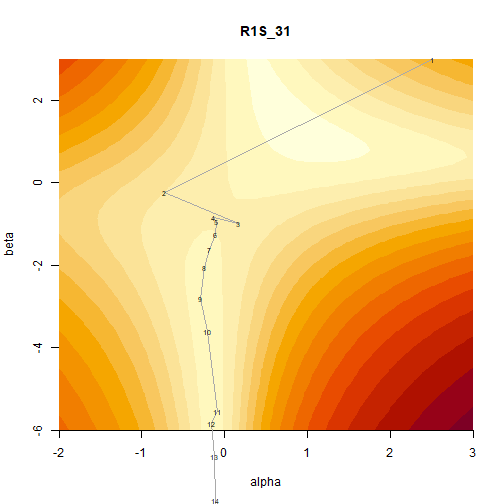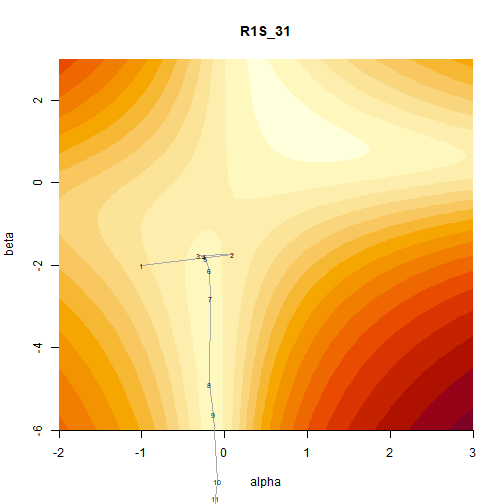Unfortunately, as we see, starting twice is not guaranteed to finish in different spots. Clearly the relation between start and end value is not so straightforward as we might like. We can be a bit more methodical about this. Let’s start at an evenly spaced number of points. We plot the starting values and a minus if the alpha goes to a negative number and a plus sign otherwise. We try this using two different optimizers, nlm which dexterMML uses and the BFGS (Broyden 1970; Fletcher 1970; Goldfarb 1970; Shanno 1970) that mirt uses by default (mirt offers a choice of different optimizers to the user, dexterMML does not).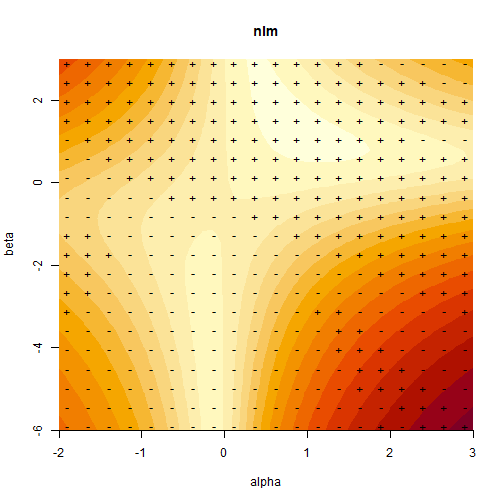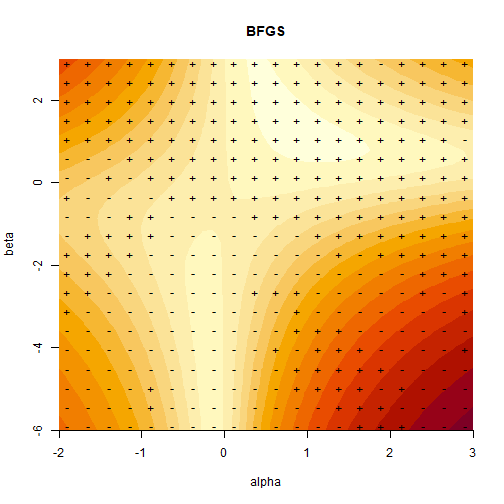That definitely fixes the problem for either algorithm, but starting in 400 different locations seems a bit excessive and will definitely slow down estimation. There are other options that could alleviate the problem. We could choose an optimizer that constrains the value of the discrimination parameter to be positive and one that constrains the value to be negative, and again compare the results. We might even constrain it to be strictly positive in this case because we inspected the TIA’s above. This scheme is possible, using the box constrained BFGS algorithm. In the present case it does give correct results. However constrained optimization is generally less reliable than unconstrained optimization, especially in higher dimensions. A different solution is to reparametrize in some way, to make the likelihood surface more amenable. Indeed, changing the itemtype option in mirt from ‘gpcmIRT’ to ‘2PL’ leads to positive alpha values for all items4.

## Conclusion

This all illustrates that it is not generally possible to have a minorization algorithm that works on any function, unless the function happens to be convex (like a 1PL). You can improve your chances by using different algorithms, different starting values or tweaking the step size. This is more art than science, and can be costly in terms of computation time. The 2PL likelihood for a single item is not a convex function, so these problems can and do sometimes occur. It is not a general failing of mirt, nor can DexterMML be considered immune to this problem. We cannot test all possible datasets after all.

So even if we cannot know whether it is completely reliable, a big advantage of dexterMML in this example is that the two item curves could be easily plotted along with what little is left of the observed data in an IRT model, namely the manifest probabilities plotted against the unobservable latent ability. “Above all else, show the data” (Tufte 1986) is an advice that should be taken to heart5.

The example above is not unique. I have seen similar problems before in other software. The usual case is an estimated positive alpha value for a truly negatively discriminating item, so the other way around from the present example. For example, a completely unrelated program also called Mirt (Glas 2010) was prescribed for use in the calibration of the end of primary education tests in the Netherlands. In this program it was impossible to get negative values for alpha parameters, even when purposefully introducing a key error in complete data. I don’t know whether this was by design, lack of awareness of the problem or a naive assumption that key-errors do not occur. However, it was used for a number of years and also did not provide any plots, so there is a possibility that negatively discriminating items have been included in (one of the) end of primary education tests in the Netherlands.

Of course, such estimation errors are relatively rare and not a cause for too much concern, depending on the circumstance. We avoid the 2PL for summative tests in any case, for wholly different and more important reasons, see e.g. here, here and here.

Broyden, C. G. 1970. “The Convergence of a Class of Double-Rank Minimization Algorithms 1. General Considerations.” IMA Journal of Applied Mathematics 6 (1): 76–90. https://doi.org/10.1093/imamat/6.1.76.
Chalmers, R. Philip. 2012. mirt: A Multidimensional Item Response Theory Package for the R Environment.” Journal of Statistical Software 48 (6): 1–29. http://www.jstatsoft.org/v48/i06/.
Dempster, A. P., N. M. Laird, and D. B. Rubin. 1977. “Maximum Likelihood from Incomplete Data via the EM Algorithm.” Journal of the Royal Statistical Society: Series B (Methodological) 39 (1): 1–22. https://doi.org/10.1111/j.2517-6161.1977.tb01600.x.
Dennis, John E., and Bobby Schnabel. 1983. “Numerical Methods for Unconstrained Optimization and Nonlinear Equations.” In Prentice Hall Series in Computational Mathematics.
Fletcher, R. 1970. “A New Approach to Variable Metric Algorithms.” The Computer Journal 13 (3): 317–22. https://doi.org/10.1093/comjnl/13.3.317.
Glas, C. A. W. 2010. Multidimensional Item Response Theory (MIRT), Manual and Computer Program.
Goldfarb, Donald. 1970. “A Family of Variable-Metric Methods Derived by Variational Means.” Mathematics of Computation 24 (109): 23–26. https://doi.org/10.1090/s0025-5718-1970-0258249-6.
Shanno, D. F. 1970. “Conditioning of Quasi-Newton Methods for Function Minimization.” Mathematics of Computation 24 (111): 647–56. https://doi.org/10.1090/s0025-5718-1970-0274029-x.
Tufte, Edward R. 1986. The Visual Display of Quantitative Information. USA: Graphics Press.

1. sometimes a few Newton-Raphson steps are taken near the end of the iterations, which do update the complete set of parameters at the same time, but this is computationally expensive in large datasets and not part of the EM iterations↩︎

2. for historic reasons, maximization is almost always done by minimizing -1 * the function to be maximized, so the technique is called minorization, and you usually have to put a minus in front of your target function to be maximized when you supply it to the algorithm. To add to the confusion, minorization and optimization are often used interchangeably↩︎

3. this is possible for convex functions, where there is necessarily only one minimum↩︎

4. ‘2PL’ would be the normal choice in mirt in this case, I had ‘gpcmIRT’ left over in my code from an earlier attempt at the reading part of the dataset, which has polytomous items. 2PL is a special case of the generalized partial credit model, with in this case possibly a different internal parametrization in mirt or it could just be estimated in a slightly different way.↩︎

5. A similar plot might be possible to make using mirt but I could not find it↩︎